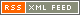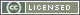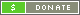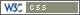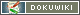Dear Brian, Jerry & The uw Group,

INTRODUCTION – Brian has asked an interesting and provocative question that gets down to some basics of a peculiar type of transmission line system (full question repeated below), “what is the VSWR and voltage on a 1/4 wave matching transformer that has a four 50 ohm loads?” As usual, there is more going on than first meets the eye.

THE MATCHING SECTION – The four loads of 50 ohms each combine to create an output load of 50/4 = 12.5 ohms. We have often been told that a single section type of 1/4 wave matching transformer should have an impedance of Square-Root(Zin x Zout). In this case that's (50 x 12.5)^0.5 = 25 ohms.

HOW DOES IT WORK? – A 1/4 wave long matching section will act like an “Impedance Inverter”. It is a 25 ohm line looking into a total load of 12.5 ohms (a 2:1 VSWR), therefore it presents an input impedance of twice it's Zo (25 ohms) = 50 ohms.

However, this is a 2:1 VSWR on a 25 ohm line, not a 50 ohm line – that makes a difference.

WHAT VOLTAGE and CURRENT? – Ordinarily, a 50 ohm line with a 2:1 VSWR would have a voltage (and current) that is 33% higher than normal [1.33 volts or amps relative] somewhere on that line, as well as 33% lower than normal [0.66 volts or amps relative] somewhere else, if it is at least 1/4 wave long, and resistively terminated. The 2:1 VSWR is the result of the 1.33/0.66 voltage (or current) ratio = 2.0.

WHAT'S THE REAL VOLTAGE – But, in this case the input voltage on the 25 ohm line will be 1.0 volts (relative), and the output voltage will be 0.5 volts relative – still a 2:1 VSWR. To be specific, if you are running a kilowatt CW on a 50 ohm line, the voltage [& current] will be 223.6 volts [4.5 amps] (rms) on your 50 ohm transmission line; there will be 223.6 volts [4.5 amps] at the input end of the your 1/4 wave 25 ohm matching transformer, and there will be 111.8 volts [8.9 amps] at the output end. The absolute magnitude of the voltage along the 25 ohm line will taper with a waveform that will look like a COSINE on a Pedestal; the upper portion will look like one half of the COSINE Wave from 0.0 degrees to 180 degrees. The waveform is illustrated in Theodore Moreno, “Microwave Transmission Design Data”, Dover Publications, 1948, p 22, Fig 2-5, figure E.

IT'S EASIER THAN YOU THINK – The bottom line of all of this is you do not have to protect the system from a high voltage condition. You only have to consider that the Current on the 25 ohm line will be twice as high at the output load end. But, that almost doesn't matter. A 25 ohm line has a fatter center conductor, and it can easily tolerate twice the current. By the way, you can synthesize a 25 ohm line by putting a pair of 1/4 wave long, 50 ohm lines, in parallel.

VOLTAGE IS NOT A PROBLEM – My good friend Hank Keen, W2CTK (sk) has made this measurement many times: Pulses of RF applied to a Type N connector with a Polonium Source nearby (a source of ions) will not arc-over until you exceed 20 kW (1,000 volts rms). Air at sea level is good for (has a Dielectric Strength of) 30 kV/cm = 76 kV/inch. Also, moisture in the air (high humidity) does not change the Dielectric Strength. Almost all connectors fail from current overheating the center pin, not from arc-over.

LOSS IS ALSO NOT A PROBLEM – Your matching transformer is only 1/4 wave long, and your connectors are much-much shorter yet. Unless you use resistive materials, or insulators that have a big “Loss Tangent”, you can only create RF loss by using transmission line materials that have a significant length. There are many mis-informed amateurs who believe each UHF Connector has 0.5 dB of loss at 432 MHz – this is crazy. Yes, they may have a 1.15:1 VSWR. This really is not a “Loss” mechanism, it a “Reflection” mechanism. The true 432 MHz insertion loss (of a good brand) is about 2 watts out of 1,000 – that's about 0.009 dB each.

73 es Good High Power DX,
Dick, K2RIW